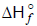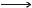Courses

MCQ (Previous Year Questions) - Thermochemistry (Level 2)

7 Questions MCQ Test | MCQ (Previous Year Questions) - Thermochemistry (Level 2)

Description
Attempt MCQ (Previous Year Questions) - Thermochemistry (Level 2) | 7 questions in 10 minutes | Mock test for JEE preparation | Free important questions MCQ to study for JEE Exam | Download free PDF with solutions
QUESTION: 1

Solution:
QUESTION: 2

Solution:
QUESTION: 3

Which of the following reactions defines?                                [JEE 2003]

Solution:
QUESTION: 4

The bond energy (in kcal mol-1) of a C - C single bond is approximately                    [JEE 2010]

Solution:
QUESTION: 5

The species which by definition has zero standard molar enthalpy of formation at 298 K is             [JEE 2010]

Solution: Some standards elements have zero standard molar enthalpy which must we should always remember ,like br2(l),cl2(g),f2(g),i2(s) and many more.
QUESTION: 6

Using the data provided, calculate the multiple bond energy (kJ mol-1) of a CC bond in C2H2. That energy is (take the bond energy of a C_H bond as 350 kJ mol-1)                                               [JEE 2012]

2C(s) + H2(g)C2H2(g) ΔH = 225 kJ mol-1

2C(s)2C(g)               ΔH = 1410 kJ mol-1

H2(g)2H(g)               ΔH = 330 kJ mol-1

Solution:
QUESTION: 7

The standard enthalpies of formation of CO2(g), H2O(l) and glucose(s) at 25ºC are -400 kJ/mol, -300 kJ/mol and -1300 kJ/mol, respectively. The standard enthalpy of combustion per gram of glucose at 25ºC is                       [JEE Advance 2013]

Solution:Use Code STAYHOME200 and get INR 200 additional OFF Use Coupon Code

Track your progress, build streaks, highlight & save important lessons and more!

Similar ContentRelated tests